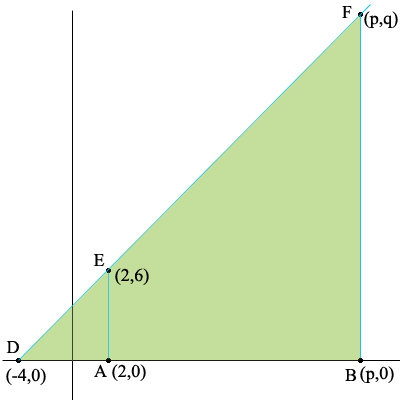SEARCH HOMEMath Central Quandaries & QueriesQuestion from Rogerson, a student: The point F is on the line through D(-4, 0) and E(2, 6) so that DF=4DE. Find the coordinates of F.Rogerson,

Suppose the point F has coordinates (p, q). I can help you get started on finding p and you can then modify what I have done to find q.

First I would draw a diagram and label it.The key here is that triangles FDB and EDA are similar. You are told that |FD|/|ED| = 4. Can you now find p?

PennyMath Central is supported by the University of Regina and The Pacific Institute for the Mathematical Sciences.